Function Repository Resource:

# VisualizeDigitPath

Get a graph of the sequential digit path of a number

Contributed by: Jordan Hasler, Nicholas Brunk and the Wolfram|Alpha Math Team
 ResourceFunction["VisualizeDigitPath"][n,len] yields a graph of the sequential digit path of the number n including exactly len digits. ResourceFunction["VisualizeDigitPath"][{n,b},len] yields a graph of the sequential digit path in base b. ResourceFunction["VisualizeDigitPath"][{n,base},len,type] returns the resulting visualization specified by type.

## Details

The edge weight is displayed in the tooltip upon Mouseover.
The option type defaults to "Multigraph". Specified types can also include "WeightedGraph" or "MatrixPlot".

## Examples

### Basic Examples (2)

Return a Graph of the digits of Pi up to the first 30 digits:

 In:=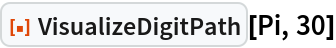Out=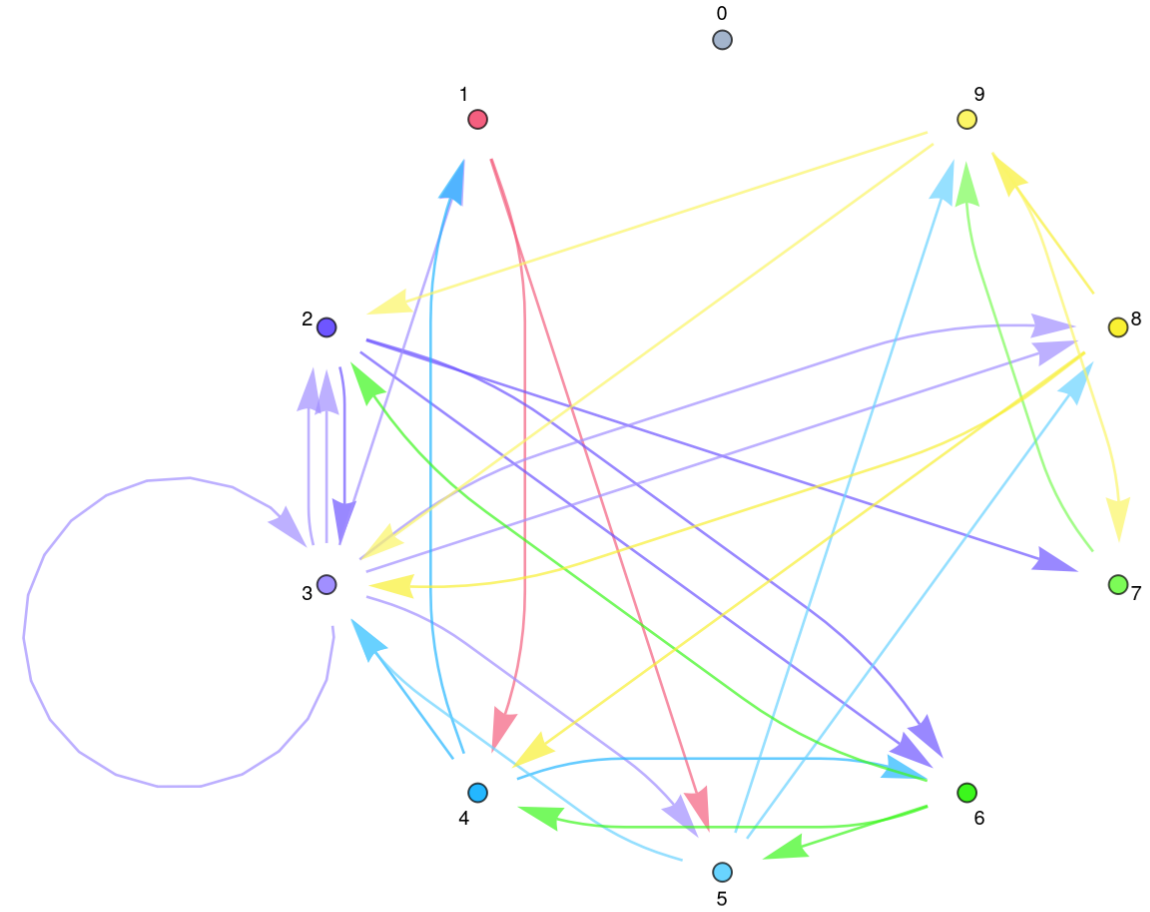Return a weighted Graph of the digits of Pi up to the first 30 digits:

 In:=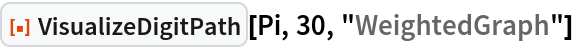Out=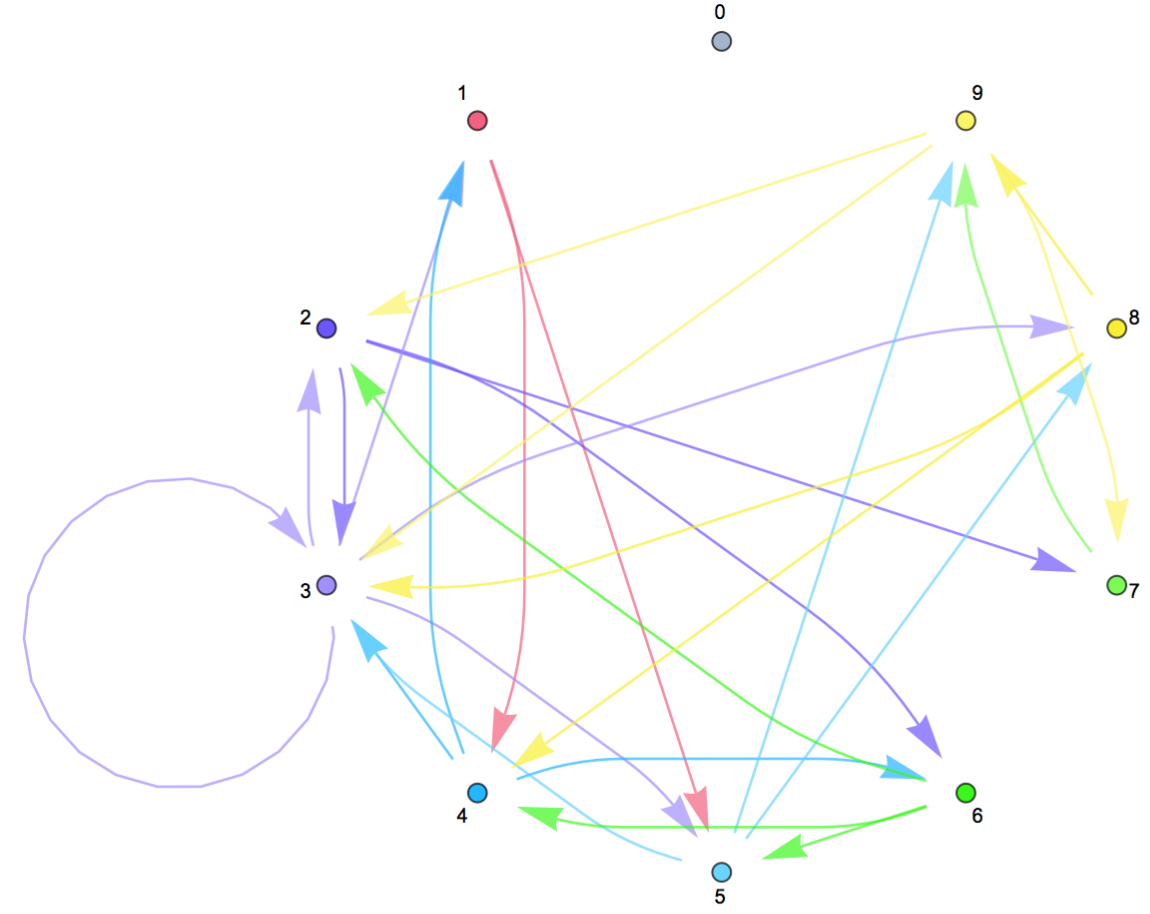### Scope (2)

Return a Graph of the digits of Pi in base 3 up to the first 15 digits:

 In:=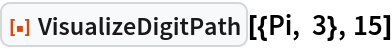Out=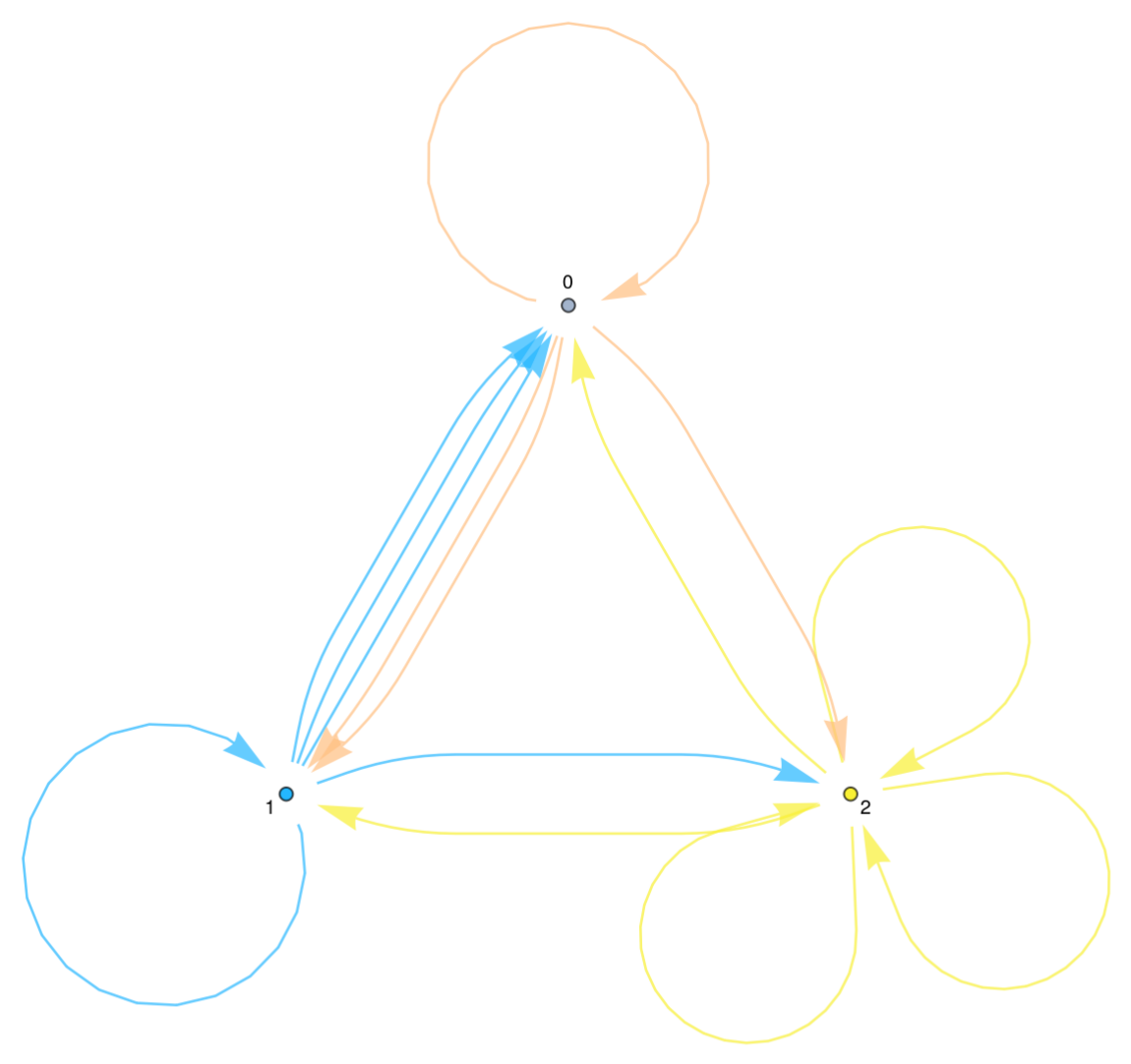Return a MatrixPlot of the digits of E up to the first 30 digits, displaying the relative frequencies of the transitions:

 In:=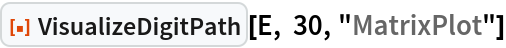Out=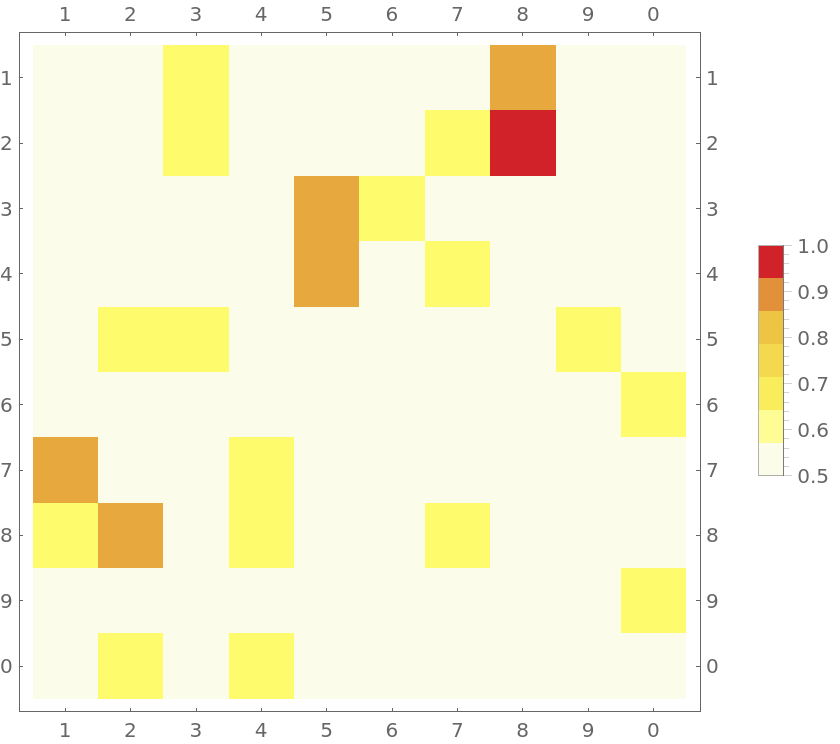### Neat Examples (1)

For a pseudorandom number, as you acquire more samples, the coefficient of variation scales to zero as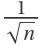, implying that the number is normal:

 In:=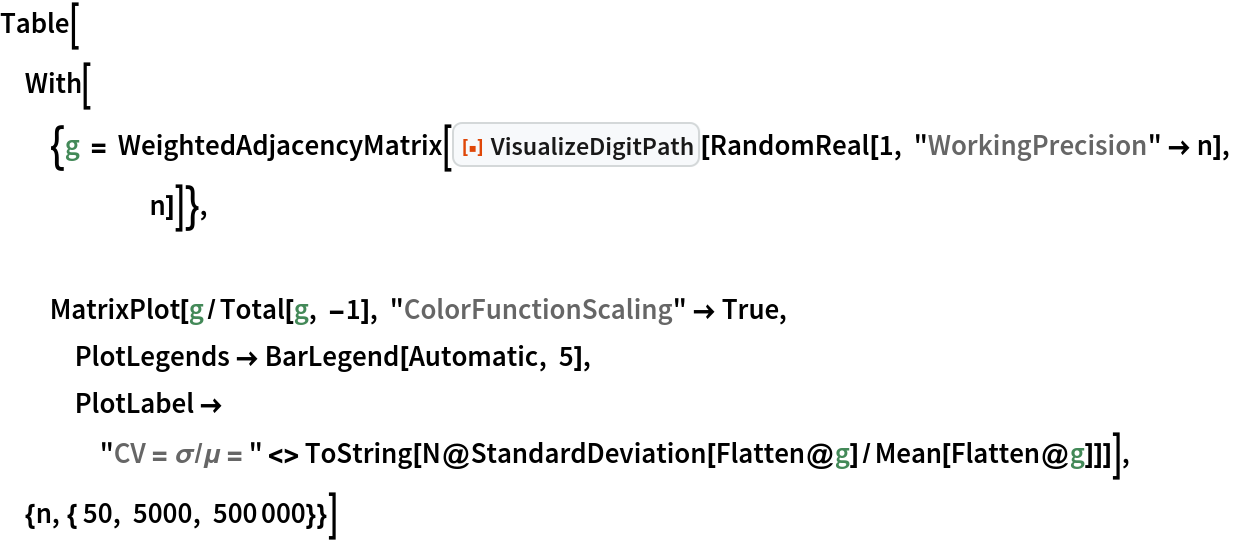Out=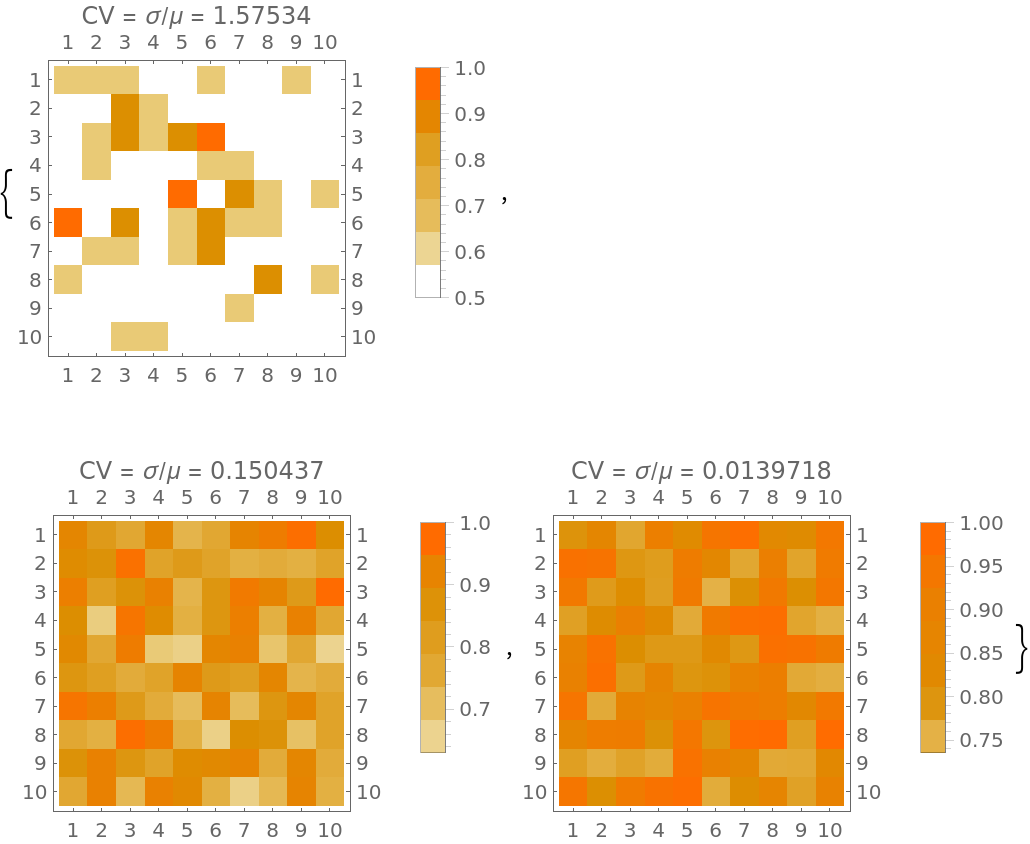## Publisher

Wolfram|Alpha Math Team

## Version History

• 2.0.0 – 23 March 2023
• 1.0.0 – 01 March 2021

## Author Notes

To view the full source code for VisualizeDigitPath, evaluate the following:

 In:=